## Sunday, 10 July 2022

### Biodiverse now calculates indices for the variation in phylogenetic distinctness

Biodiverse has included calculations of indices from the phylocom system for several versions, specifically the Mean Phylogenetic Distance (MPD) and Mean Nearest Taxon Distance (MNTD).  The MPD is the average of the pair-wise distances between tree tips in a sample, where the distances pass through all the shared ancestors below the most recent common ancestor.  The MNTD is the average distance for each tip to its nearest tip in the sample.

There are many ways of slicing and diving a sample, and one of the development principles of Biodiverse is to provide more details rather than less.  Consequently there are also indices for the pair-wise root mean standard deviation (RMSD), minimum and maximum distances between a sample of tips on a tree.

The min and max are simply the longest and shortest distances in the pairwise sample, so the distances between the most and least related pairs.  The RMSD is the square root of the mean squared distance and is a measure of the variability in a sample. It is analogous to a standard deviation but where the expected value (the mean) is zero, and follows the same formulation as the Root Mean Squared Error except a value of zero in RMSE means no error whereas in RMSD it means a zero distance between tips on the tree.

However, the RMSD is not the variance and sometimes one is looking to see how a set of pair-wise distances is distributed around the mean.  This is where the Variance becomes useful, as first described by Warwick and Clarke (2001).

Biodiverse version 4 includes indices for the variance of the pairwise distances.  The index names are subject to change before then but for now follow the pattern PMPD1_VARIANCE, PMPD2_VARIANCE and PMPD2_VARIANCE, where the 1, 2 and 3 indicate unweighted (each tip counts equally), locally range weighted (tips count as many groups they occur in the neighbourhood) and locally abundance weighted (using the number of samples of each tip in the neighbourhood).  These are calculated by default when the relevant MPS and MNTD indices are requested.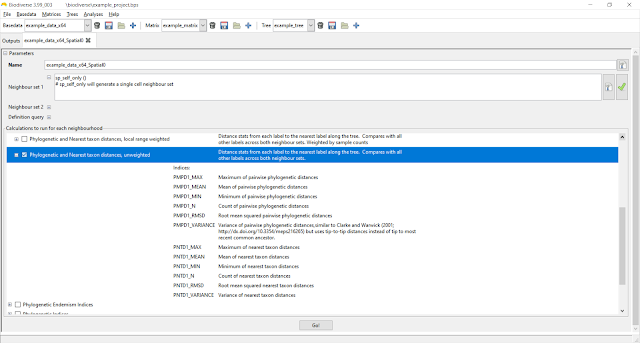The variance indices are calculated with the other MPD and MNTD indices.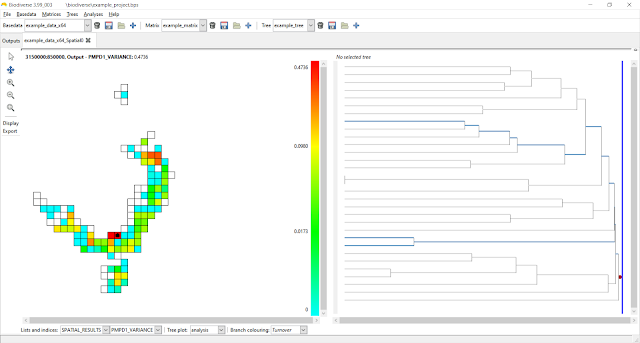Plotting is the same as for any index.  Some cells are blank because values are undefined when the sample contains only one tip, and therefore no path between tips.  Zero variances are where there are only two tips, and thus no variation.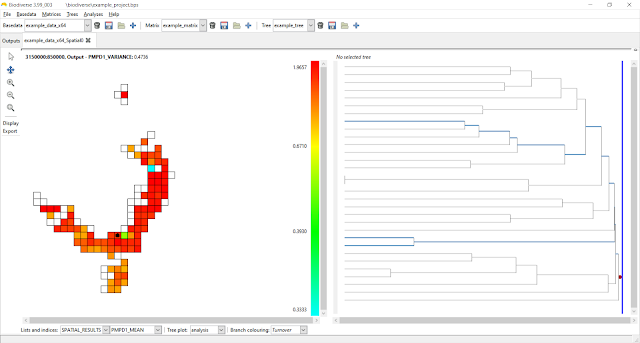This is just a plot of the mean for comparison.

### But are the values significant?

A common approach to testing significance of the MPD and MNTD indices in the unweighted case is to use a resampling approach.  For each sample this generates a distribution of possible values under random resampling of the same number of tips.  More details are given in another blog post.

The unweighted pairwise variance is also assessed in this way, with the index name using NET_VPD.  As with NRI and NTI, this is a z-score so values more extreme than +/-1.96 can be considered significantly higher or lower than expected.

The resampling approach uses the same code as for NRI and NTI so the same sequence of resamples can be used across NRI, NTI and NET_VPD, although in Biodiverse version 4 this is only for NTI for non-ultrametric trees an exact calculation is used for NRI with any trees and for NTI for ultrametric trees.  This exact calculation avoids resampling and is much faster to run.  More details and references are in the same blog post referred to above).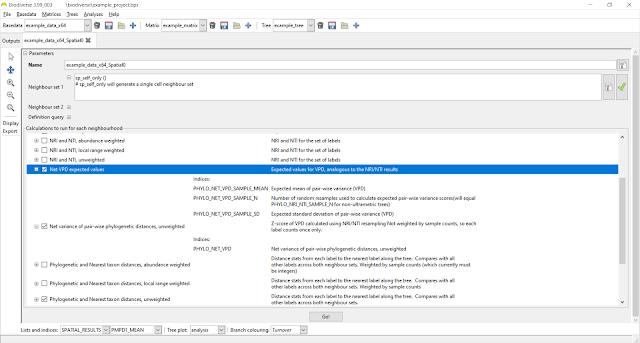The NET_VPD indices are also under the PhyloCom set.  Users can calculate the NET_VPD as well as the expected values used in its calculation.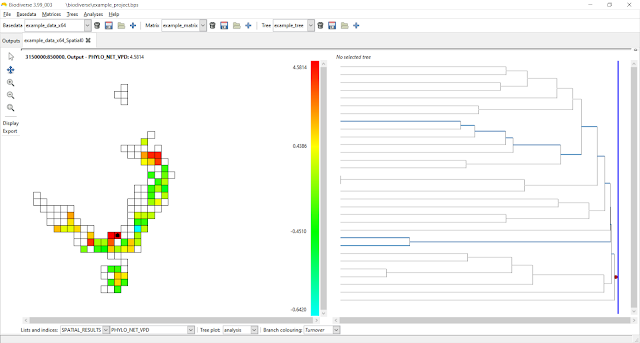Values are z-scores.  At least three tips are needed to calculate the z-score as standard deviations are always zero for two tips and thus the z-score is undefined.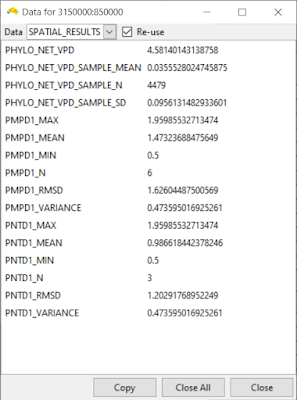Control clicking on cells allow users to see the values for all indices that were calculated (within each output list, where SPATIAL_RESULTS is where most go).

Shawn Laffan

10-Jul-2022

For more details about Biodiverse, see http://shawnlaffan.github.io/biodiverse/

To see what else Biodiverse has been used for, see https://github.com/shawnlaffan/biodiverse/wiki/PublicationsList

You can also join the Biodiverse-users mailing list at https://groups.google.com/group/Biodiverse-users or start a discussion at https://github.com/shawnlaffan/biodiverse/discussions